# 🌱 Basics

## Getting started

EOmaps is all about `Maps` objects.

The first `Maps` object that is created will initialize a matplotlib.Figure and a cartopy.GeoAxes for a map.

```from eomaps import Maps
m = Maps(crs=4326,               # create a Maps-object with a map in lon/lat (epsg=4326) projection
layer="first layer",         # assign the layer "first_layer"
figsize=(7, 5))              # set the figure-size to 7x5
m.set_extent((-25, 35, 25, 70))  # set the extent of the map
```
• `crs` represents the projection used for plotting

• `layer` represents the name of the layer associated with the Maps-object (see Layer management).

• all additional keyword arguments are forwarded to the creation of the matplotlib-figure (e.g.: `figsize`, `frameon`, `edgecolor` etc).

Possible ways for specifying the `crs` for plotting are:

• If you provide an integer, it is identified as an epsg-code (e.g. `4326`, `3035`, etc.)

• 4326 defaults to PlateCarree projection

• All other CRS usable for plotting are accessible via `Maps.CRS`, e.g.: `crs=Maps.CRS.Orthographic()`, `crs=Maps.CRS.Equi7_EU`

• `Maps.CRS` is just an accessor for `cartopy.crs`

• For a full list of available projections see: Cartopy projections

 `Maps` The base-class for generating plots with EOmaps. `Maps.CRS` The crs module defines Coordinate Reference Systems and the transformations between them. `Maps.set_extent` Set the extent (x0, x1, y0, y1) of the map in the given coordinate system. `Maps.add_title` Convenience function to add a title to the map.

## Layer management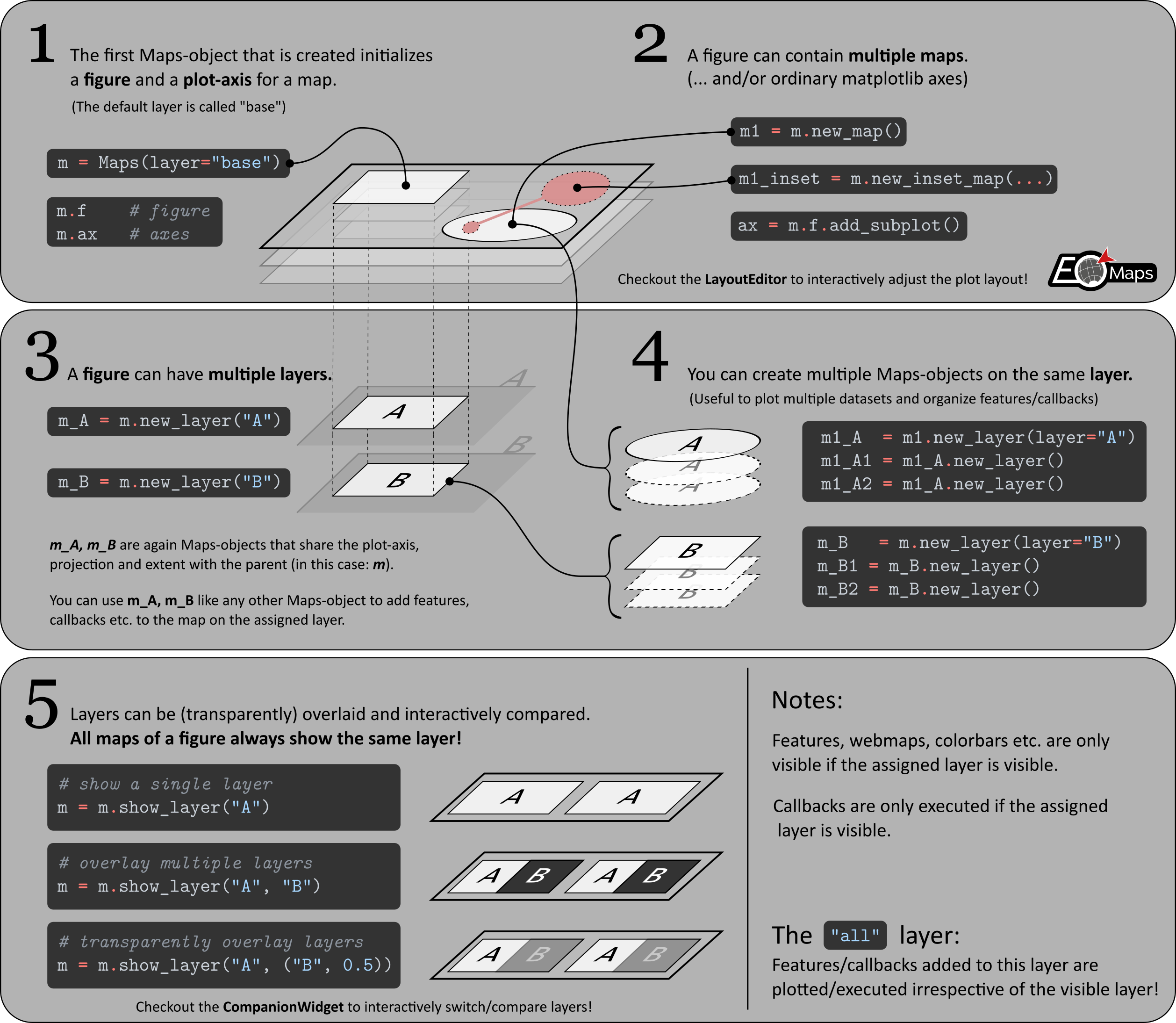A `Maps` object represents a collection of features, callbacks,.. on the assigned layer.

Once you have created a map, you can create additional `Maps` objects for the same map by using `Maps.new_layer()`.

🌱 If no explicit layer-name is provided, the returned `Maps` object will use the same layer as the parent `Maps` object.

• This is especially useful if you want to plot multiple datasets on the same map and layer.

🌱 To create a NEW layer named `"my_layer"`, use `m2 = m.new_layer("my_layer")`

```from eomaps import Maps
m = Maps()                           # same as `m = Maps(crs=4326, layer="base")`

m_ocean = m.new_layer(layer="ocean") # create a new layer named "ocean"
m_ocean.add_feature.preset.ocean()   # features on this layer will only be visible if the "ocean" layer is visible!

m_ocean2 = m_ocean.new_layer()       # "m_ocean2" is just another Maps-object on the same layer as "m_ocean"!
m_ocean2.set_data(                   # assign a dataset to this Maps-object
data=[.14,.25,.38],
x=[1,2,3], y=[3,5,7],
crs=4326)
m_ocean2.set_shape.ellipses()        # set the shape that is used to represent the datapoints
m_ocean2.plot_map()                  # plot the data

m.show_layer("ocean")                # show the "ocean" layer
m.util.layer_selector()              # get a utility widget to quickly switch between existing layers
```

The “all” layer

There is one layer-name that has a special meaning… the `"all"` layer.
Any callbacks and features added to this layer will be executed on ALL other layers as well!

You can add features and callbacks to the `all` layer via:

• using the shortcut `m.all. ...`

• creating a dedicated `Maps` object via `m_all = Maps(layer="all")` or `m_all = m.new_layer("all")`

• using the “layer” kwarg of functions e.g. `m.plot_map(layer="all")`

```from eomaps import Maps
m = Maps()

m_ocean = m.new_layer(layer="ocean") # create a new layer named "ocean"
m.show_layer("ocean")                # show the "ocean" layer (note that it has coastlines as well!)
```

Artists added with methods outside of EOmaps

If you use methods that are NOT provided by EOmaps, the corresponding artists will always appear on the `"base"` layer by default! (e.g. `cartopy` or `matplotlib` methods accessible via `m.ax.` or `m.f.` like `m.ax.plot(...)`)

In most cases this behavior is sufficient… for more complicated use-cases, artists must be explicitly added to the Blit Manager (`m.BM`) so that `EOmaps` can handle drawing accordingly.

To put the artists on dedicated layers, use one of the the following options:

• For artists that are dynamically updated on each event, use `m.BM.add_artist(artist, layer=...)`

• For “background” artists that only require updates on pan/zoom/resize, use `m.BM.add_bg_artist(artist, layer=...)`

```from eomaps import Maps
m = Maps()

# draw a red X over the whole axis and put the lines
# as background-artists on the layer "mylayer"
(l1, ) = m.ax.plot([0, 1], [0, 1], lw=5, c="r", transform=m.ax.transAxes)
(l2, ) = m.ax.plot([0, 1], [1, 0], lw=5, c="r", transform=m.ax.transAxes)

m.show_layer("mylayer")
```

### 🗗 Combine & compare multiple layers

Using the 🧰 Companion Widget

Usually it is most convenient to combine and compare layers via the 🧰 Companion Widget.

• Use the dropdown-list at the top-right to select a single layer or overlay multiple layers.

• Click on a single layer to make it the visible layer.

• Hold down `control` or `shift` to overlay multiple layers.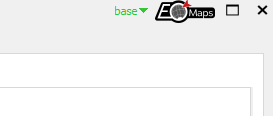• Select one or more layers to dynamically adjust the stacking-order via the layer-tabs of the Compare and Edit views.

• Hold down `control` while clicking on a tab to make it the visible layer.

• Hold down `shift` while clicking on a tab to overlay multiple layers.

• Re-arrange the tabs to change the stacking-order of the layers.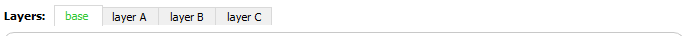To programmatically switch between layers or view a layer that represents a combination of multiple existing layers, use `Maps.show_layer()`.

🌱 If you provide a single layer-name, the map will show the corresponding layer, e.g. `m.show_layer("my_layer")`

🌱 To (transparently) overlay multiple existing layers, use one of the following options:

• Provide multiple layer names or tuples of the form `(< layer-name >, < transparency [0-1] >)`

• `m.show_layer("A", "B")` will overlay all features of the layer `B` on top of the layer `A`.

• `m.show_layer("A", ("B", 0.5))` will overlay the layer `B` with 50% transparency on top of the layer `A`.

• Provide a combined layer name by separating the individual layer names you want to show with a `"|"` character.

• `m.show_layer("A|B")` will overlay all features of the layer `B` on top of the layer `A`.

• To transparently overlay a layer, add the transparency to the layer-name in curly-brackets, e.g.: `"<layer-name>{<transparency>}"`.

• `m.show_layer("A|B{0.5}")` will overlay the layer `B` with 50% transparency on top of the layer `A`.

```from eomaps import Maps
m = Maps(layer="first")

m2 = m.new_layer("second")                # create a new layer and plot some data
m2.set_data(data=[.14,.25,.38],
x=[10,20,30], y=[30,50,70],
crs=4326)
m2.plot_map(zorder=1)                     # plot the data "below" the ocean

m.show_layer("first", ("second", .75))   # overlay the second layer with 25% transparency
```

🌱 If you want to overlay a part of the screen with a different layer, have a look at `peek_layer()` callbacks**!

 `peek_layer` Overlay a part of the map with a different layer if you click on the map.
```from eomaps import Maps
m = Maps()

m.cb.click.attach.peek_layer(layer=["ocean", ("land", 0.5)], shape="round", how=0.4)
```

The “stacking order” of features and layers

The stacking order of features at the same layer is controlled by the `zorder` argument.

• e.g. `m.plot_map(zorder=1)` or `m.add_feature.cultural.urban_areas(zorder=10)`

If you stack multiple layers on top of each other, the stacking is determined by the order of the layer-names (from right to left)

• e.g. `m.show_layer("A", "B")` will show the layer `"B"` on top of the layer `"A"`

• you can stack as many layers as you like! `m.show_layer("A", "B", ("C", 0.5), "D", ...)`

 `Maps.new_layer` Create a new Maps-object that shares the same plot-axes. `Maps.all` Get a Maps-object on the "all" layer. `Maps.show` Show the map (only required for non-interactive matplotlib backends). `Maps.show_layer` Show a single layer or (transparently) overlay multiple selected layers. `Maps.fetch_layers` Fetch (and cache) the layers of a map.

## Image export (jpeg, png, svg, etc.)

Once the map is ready, an image of the map can be saved at any time by using `Maps.savefig()`

```from eomaps import Maps
m = Maps()
m.savefig("snapshot1.png", dpi=200, transparent=True)
```

To adjust the margins of the subplots, use `m.subplots_adjust()`, or have a look at the 🏗️ Layout Editor!

```from eomaps import Maps
m = Maps()
```

Export to clipboard (`ctrl + c`)

If you use `PyQt5` as matplotlib-backend, you can also press (`control` + `c`) to export the figure to the clipboard.

The export will be performed using the currently set export-parameters in the 🧰 Companion Widget . Alternatively, you can also programmatically set the export-parameters via `Maps.set_clipboard_kwargs()` .

Notes on exporting high-dpi figures

EOmaps tries its best to follow the WYSIWYG concept (e.g. “What You See Is What You Get”). However, if you export the map with a dpi-value other than `100`, there are certain circumstances where the final image might look different. To summarize:

• Changing the dpi of the figure requires a re-draw of all plotted datasets.

• if you use `shade` shapes to represent the data, using a higher dpi-value can result in a very different appearance of the data!

• WebMap services usually come as image-tiles with 96 dpi

• by default, images are not re-fetched when saving the map to keep the original appearance

• If you want to re-fetch the WebMap based on the export-dpi, use `m.savefig(refetch_wms=True)`.

• Note: increasing the dpi will result in an increase in the number of tiles that have to be fetched. If the number of required tiles is too large, the server might reject the request and the map might have gaps or no tiles at all.

 `Maps.savefig` Save the current figure. `Maps.subplots_adjust` Update the subplot parameters of the grid. `Maps.set_clipboard_kwargs` Set GLOBAL savefig parameters for all Maps objects on export to the clipboard.

## Multiple Maps (and/or plots) in one figure

It is possible to combine multiple `EOmaps` maps and/or ordinary `matpltolib` plots in one figure.

The figure used by a `Maps` object is set via the `f` argument, e.g.: `m = Maps(f=...)`. If no figure is provided, a new figure is created whenever you initialize a `Maps` object. The figure-instance of an existing `Maps` object is accessible via `m.f`

• To add a map to an existing figure, use `m2 = m.new_map()` (requires EOmaps >= v6.1) or pass the figure-instance on initialization of a new `Maps` object.

• To add a ordinary `matplotlib` plot to a figure containing an eomaps-map, use `m.f.add_subplot()` or `m.f.add_axes()`.

The initial position of the axes used by a `Maps` object is set via the `ax` argument, e.g.: `m = Maps(ax=...)` or `m2 = m.new_map(ax=...)`

• The syntax for positioning axes is similar to matplotlibs `f.add_subplot()` or `f.add_axes()`

• The axis-instance of an existing `Maps` object is accessible via `m.ax`

Note

Make sure to have a look at the 🏗️ Layout Editor on how to re-position and re-scale axes to arbitrary positions!

 `Maps` The base-class for generating plots with EOmaps. `Maps.new_map` Create a new map that shares the figure with this Maps-object.

In the following, the most commonly used cases are introduced:

### Grid positioning

To position the map in a (virtual) grid, one of the following options are possible:

• Three integers `(nrows, ncols, index)` (or 2 integers and a tuple).

• The map will take the `index` position on a grid with `nrows` rows and `ncols` columns.

• `index` starts at 1 in the upper left corner and increases to the right.

• `index` can also be a two-tuple specifying the (first, last) indices (1-based, and including last) of the map, e.g., `Maps(ax=(3, 1, (1, 2)))` makes a map that spans the upper 2/3 of the figure.

 ```from eomaps import Maps # ----- initialize a figure with an EOmaps map # position = item 1 of a 2x1 grid m = Maps(ax=(2, 1, 1)) # ----- add a normal matplotlib axes # position = item 2 of a 2x1 grid ax = m.f.add_subplot(2, 1, 2) ```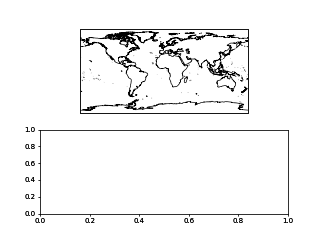```from eomaps import Maps # ----- initialize a figure with an EOmaps map # position = item 1 of a 2x2 grid m = Maps(ax=(2, 2, 1)) # ----- add another Map to the same figure # position = item 3 of a 2x2 grid m2 = m.new_map(ax=(2, 2, 3)) # ----- add a normal matplotlib axes # position = second item of a 1x2 grid ax = m.f.add_subplot(1, 2, 2) ```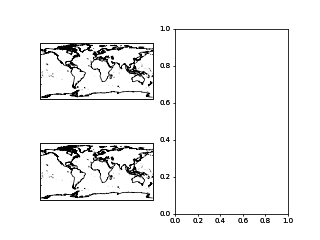```from eomaps import Maps # ----- initialize a figure with an EOmaps map # position = span 2 rows of a 3x1 grid m = Maps(ax=(3, 1, (1, 2))) # ----- add a normal matplotlib axes # position = item 3 of a 3x1 grid ax = m.f.add_subplot(3, 1, 3) ```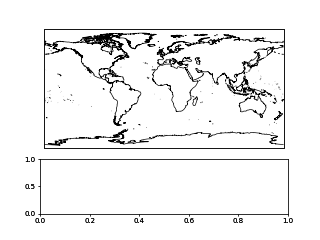• A 3-digit integer.

• The digits are interpreted as if given separately as three single-digit integers, i.e. `Maps(ax=235)` is the same as `Maps(ax=(2, 3, 5))`.

• Note that this can only be used if there are no more than 9 subplots.

 ```from eomaps import Maps # ----- initialize a figure with an EOmaps map m = Maps(ax=211) # ----- add a normal matplotlib axes ax = m.f.add_subplot(212) ```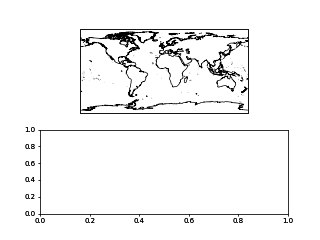```from eomaps import Maps # ----- initialize a figure with an EOmaps map m = Maps(ax=221) # ----- add 2 more Maps to the same figure m2 = m.new_map(ax=222) m3 = m.new_map(ax=223) # ----- add a normal matplotlib axes ax = m.f.add_subplot(224) ```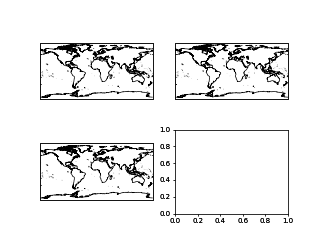```from matplotlib.gridspec import GridSpec from eomaps import Maps gs = GridSpec(2, 2) m = Maps(ax=gs[0,0]) m2 = m.new_map(ax=gs[0,1]) ax = m.f.add_subplot(gs[1,:]) ```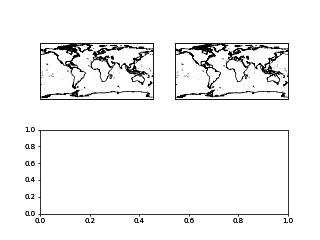### Absolute positioning

To set the absolute position of the map, provide a list of 4 floats representing `(left, bottom, width, height)`.

• The absolute position of the map expressed in relative figure coordinates (e.g. ranging from 0 to 1)

Note

Since the effective size of the Map is dependent on the current zoom-region, the position always represents the maximal area that can be occupied by the map!

Also, using `m.f.tight_layout()` will not work with axes added in this way.

 ```from eomaps import Maps # ----- initialize a figure with an EOmaps map m = Maps(ax=(.07, 0.53, .6, .3)) # ----- add a normal matplotlib axes ax = m.f.add_axes((.35, .15, .6, .2)) ```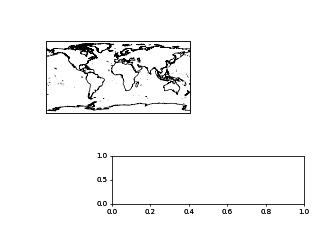### Using already existing figures / axes

It is also possible to insert an EOmaps map into an existing figure or re-use an existing axes.

• To put a map on an existing figure, provide the figure-instance via `m = Maps(f= <the figure instance>)`

• To use an existing axes, provide the axes-instance via `m = Maps(ax= <the axes instance>)`

• NOTE: The axes MUST be a cartopy-`GeoAxes`!

```import matplotlib.pyplot as plt
import cartopy
from eomaps import Maps

f = plt.figure(figsize=(10, 7))

m = Maps(f=f, ax=ax)
```

### Dynamic updates of plots in the same figure

As soon as a `Maps`-object is attached to a figure, EOmaps will handle re-drawing of the figure! Therefore dynamically updated artists must be added to the “blit-manager” (`m.BM`) to ensure that they are correctly updated.

• use `m.BM.add_artist(artist, layer=...)` if the artist should be re-drawn on any event in the figure

• use `m.BM.add_bg_artist(artist, layer=...)` if the artist should only be re-drawn if the extent of the map changes

Note

In most cases it is sufficient to simply add the whole axes-object as artist via `m.BM.add_artist(...)`.

This ensures that all artists of the axes are updated as well!

Here’s an example to show how it works:

 ```from eomaps import Maps # Initialize a new figure with an EOmaps map m = Maps(ax=223) m.ax.set_title("click me!") m.add_feature.preset.coastline() m.cb.click.attach.mark(radius=20, fc="none", ec="r", lw=2) # Add another map to the figure m2 = m.new_map(ax=224, crs=Maps.CRS.Mollweide()) m2.add_feature.preset.coastline() m2.add_feature.preset.ocean() m2.cb.click.attach.mark(radius=20, fc="none", ec="r", lw=2, n=200) # Add a "normal" matplotlib plot to the figure ax = m.f.add_subplot(211) # Since we want to dynamically update the data on the axis, it must be # added to the BlitManager to ensure that the artists are properly updated. # (EOmaps handles interactive re-drawing of the figure) m.BM.add_artist(ax, layer=m.layer) # plot some static data on the axis ax.plot([10, 20, 30, 40, 50], [10, 20, 30, 40, 50]) # define a callback that plots markers on the axis if you click on the map def cb(pos, **kwargs): ax.plot(*pos, marker="o") m.cb.click.attach(cb) # attach the callback to the first map m.cb.click.share_events(m2) # share click events between the 2 maps ```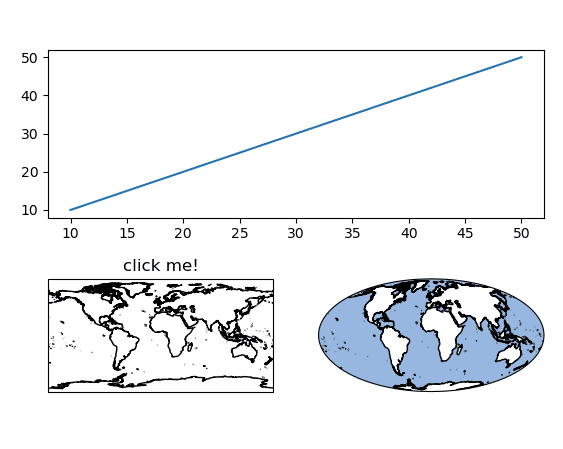### MapsGrid objects

`MapsGrid` objects can be used to create (and manage) multiple maps in one figure.

Note

While `MapsGrid` objects provide some convenience, starting with EOmaps v6.x, the preferred way of combining multiple maps and/or matplotlib axes in a figure is by using one of the options presented in the previous sections!

A `MapsGrid` creates a grid of `Maps` objects (and/or ordinary `matpltolib` axes), and provides convenience-functions to perform actions on all maps of the figure.

```from eomaps import MapsGrid
mg = MapsGrid(r=2, c=2, crs=4326)
# you can then access the individual Maps-objects via:

m_0_0_ocean = mg.m_0_0.new_layer("ocean")

# functions executed on MapsGrid objects will be executed on all Maps-objects:

# to perform more complex actions on all Maps-objects, simply loop over the MapsGrid object
for m in mg:

# set the margins of the plot-grid
mg.subplots_adjust(left=0.1, right=0.9, bottom=0.05, top=0.95, hspace=0.1, wspace=0.05)
```

Make sure to checkout the 🏗️ Layout Editor which greatly simplifies the arrangement of multiple axes within a figure!

#### Custom grids and mixed axes

Fully customized grid-definitions can be specified by providing `m_inits` and/or `ax_inits` dictionaries of the following structure:

• The keys of the dictionary are used to identify the objects

• The values of the dictionary are used to identify the position of the associated axes

• The position can be either an integer `N`, a tuple of integers or slices `(row, col)`

• Axes that span over multiple rows or columns, can be specified via `slice(start, stop)`

```dict(
name1 = N  # position the axis at the Nth grid cell (counting firs)
name2 = (row, col), # position the axis at the (row, col) grid-cell
name3 = (row, slice(col_start, col_end)) # span the axis over multiple columns
name4 = (slice(row_start, row_end), col) # span the axis over multiple rows
)
```
• `m_inits` is used to initialize `Maps` objects

• `ax_inits` is used to initialize ordinary `matplotlib` axes

The individual `Maps` objects and `matpltolib-Axes` are then accessible via:

```from eomaps import MapsGrid
mg = MapsGrid(2, 3,
m_inits=dict(ocean=(0, 0), land=(0, 2)),
ax_inits=dict(someplot=(1, slice(0, 3)))
)
# Maps object with the name "left"
# the Maps object with the name "right"

# the ordinary matplotlib-axis with the name "someplot"
mg.ax_someplot.plot([1,2,3], marker="o")
```

❗ NOTE: if `m_inits` and/or `ax_inits` are provided, ONLY the explicitly defined objects are initialized!

• The initialization of the axes is based on matplotlib’s GridSpec functionality. All additional keyword-arguments (`width_ratios, height_ratios, etc.`) are passed to the initialization of the `GridSpec` object.

• To specify unique `crs` for each `Maps` object, provide a dictionary of `crs` specifications.

```from eomaps import MapsGrid
# initialize a grid with 2 Maps objects and 1 ordinary matplotlib axes
mg = MapsGrid(2, 2,
m_inits=dict(top_row=(0, slice(0, 2)),
bottom_left=(1, 0)),
crs=dict(top_row=4326,
bottom_left=3857),
ax_inits=dict(bottom_right=(1, 1)),
width_ratios=(1, 2),
height_ratios=(2, 1))

# a map extending over the entire top-row of the grid (in epsg=4326)

# a map in the bottom left corner of the grid (in epsg=3857)

# an ordinary matplotlib axes in the bottom right corner of the grid
mg.ax_bottom_right.plot([1, 2, 3], marker="o")
```
 `MapsGrid` Initialize a grid of Maps objects `MapsGrid.join_limits` Join axis limits between all Maps objects of the grid (only possible if all maps share the same crs!) `MapsGrid.share_click_events` Share click events between all Maps objects of the grid `MapsGrid.share_pick_events` Share pick events between all Maps objects of the grid `MapsGrid.set_data` This will execute the corresponding action on ALL Maps objects of the MapsGrid! `MapsGrid.set_classify_specs` This will execute the corresponding action on ALL Maps objects of the MapsGrid! `MapsGrid.add_wms` A collection of open-access WebMap services that can be added to the maps. `MapsGrid.add_feature` Interface to the feature-layers provided by NaturalEarth. `MapsGrid.add_annotation` This will execute the corresponding action on ALL Maps objects of the MapsGrid! `MapsGrid.add_marker` This will execute the corresponding action on ALL Maps objects of the MapsGrid! `MapsGrid.add_gdf` This will execute the corresponding action on ALL Maps objects of the MapsGrid!

## Naming conventions and autocompletion

The goal of EOmaps is to provide a comprehensive, yet easy-to-use interface.

To avoid having to remember a lot of names, a concise naming-convention is applied so that autocompletion can quickly narrow-down the search to relevant functions and properties.

Once a few basics keywords have been remembered, finding the right functions and properties should be quick and easy.

Note

EOmaps works best in conjunction with “dynamic autocompletion”, e.g. by using an interactive console where you instantiate a `Maps` object first and then access dynamically updated properties and docstrings on the object.

To clarify:

• First, execute `m = Maps()` in an interactive console

• then (inside the console, not inside the editor!) use autocompletion on `m.` to get autocompletion for dynamically updated attributes.

For example the following accessors only work properly after the `Maps` object has been initialized

• `Maps.add_wms` browse available WebMap services

• `Maps.set_classify` browse available classification schemes

The following list provides an overview of the naming-conventions used within EOmaps:

All functions that add features to a map start with `add_`, e.g.:

• `Maps.add_feature`, `Maps.add_wms`, `Maps.add_annotation()`, `Maps.add_marker()`, `Maps.add_gdf()`, …

WebMap services (e.g. `Maps.add_wms`) are fetched dynamically from the respective APIs. Therefore the structure can vary from one WMS to another. The used convention is the following:

• You can navigate into the structure of the API by using “dot-access” continuously

• once you reach a level that provides layers that can be added to the map, the `.add_layer.` directive will be visible

• any `<LAYER>` returned by `.add_layer.<LAYER>` can be added to the map by simply calling it, e.g.:

• `m.add_wms.OpenStreetMap.add_layer.default()`

• `m.add_wms.OpenStreetMap.OSM_mundialis.add_layer.OSM_WMS()`

### Set data specifications - “m.set_”

All functions that set properties of the associated dataset start with `set_`, e.g.:

• `Maps.set_data()`, `Maps.set_classify`, `Maps.set_shape`, …

### Create new Maps-objects - “m.new_”

Actions that result in a new `Maps` objects start with `new_`.

• `Maps.new_layer()`, `Maps.new_inset_map()`, …

### Callbacks - “m.cb.”

Everything related to callbacks is grouped under the `cb` accessor.

• use `m.cb.<METHOD>.attach.<CALLBACK>()` to attach pre-defined callbacks

• `<METHOD>` hereby can be one of `click`, `pick` or `keypress` (but there’s no need to remember since autocompletion will do the job!).

• use `m.cb.<METHOD>.attach(custom_cb)` to attach a custom callback